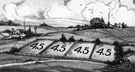# decimal

(redirected from Decimal numeral system)
Also found in: Thesaurus, Encyclopedia.
Related to Decimal numeral system: decimal number system, Hexadecimal numeral system

## dec·i·mal

(dĕs′ə-məl)
n.
1.
a. A linear array of digits that contains a single period, called the decimal point, is possibly preceded by a minus sign (-), and represents a real number, with each successive digit to the right of the decimal point indicating a multiple of the next negative power of 10, and each successive digit to the left of the decimal point indicating a multiple of the next non-negative power of ten, beginning with 100 = 1. For example, 245.3 represents the real number (2 × 102) + (4 × 101) + (5 × 100) + (3 × 10-1) = 200 + 40 + 5 + 3/10 , and -1.04 represents the real number (-1 × 100) + (-4 × 10-2). Also called mixed decimal.
b. Such an array of digits in which there are no nonzero digits to the left of the decimal point, representing a real number between -1 and 1. Also called decimal fraction.
2. A number written using the base 10.
1. Expressed or expressible as a decimal.
2.
a. Based on 10.
b. Numbered or ordered by groups of 10.

[Medieval Latin decimālis, of tenths or tithes, from Latin decima, a tenth part or tithe, from decem, ten; see dekm̥ in Indo-European roots.]

## decimal

(ˈdɛsɪməl)
n
1. (Mathematics) Also called: decimal fraction a fraction that has a denominator of a power of ten, the power depending on or deciding the decimal place. It is indicated by a decimal point to the left of the numerator, the denominator being omitted. Zeros are inserted between the point and the numerator, if necessary, to obtain the correct decimal place
2. (Mathematics) any number used in the decimal system
3. (Mathematics)
a. relating to or using powers of ten
b. of the base ten
4. (Mathematics) (prenominal) expressed as a decimal
[C17: from Medieval Latin decimālis of tithes, from Latin decima a tenth, from decem ten]

## dec•i•mal

(ˈdɛs ə məl, ˈdɛs məl)

1. pertaining to tenths or to the number 10.
2. proceeding by tens: a decimal system.
n.
[1600–10; < Medieval Latin decimālis of tenths = Latin decim(a) tenth (derivative of decem ten) + -ālis -al1]

## dec·i·mal

(dĕs′ə-məl)
1. A representation of a real number using the base ten and decimal notation, such as 201.4, 3.89, or 0.0006.
2. A decimal having no digits to the left of the decimal point except zero, such as 0.2 or 0.00354.
ThesaurusAntonymsRelated WordsSynonymsLegend:
 Noun 1decimal - a proper fraction whose denominator is a power of 10decimal fractionproper fraction - a fraction with a numerator smaller than the denominatorcirculating decimal, recurring decimal, repeating decimal - a decimal with a sequence of digits that repeats itself indefinitely 2 decimal - a number in the decimal systemnumber - a concept of quantity involving zero and units; "every number has a unique position in the sequence" Adj. 1 decimal - numbered or proceeding by tens; based on ten; "the decimal system"denaryquantitative - expressible as a quantity or relating to or susceptible of measurement; "export wheat without quantitative limitations"; "quantitative analysis determines the amounts and proportions of the chemical constituents of a substance or mixture"
Translations
عُشْريعُشْرِيّ
decimal
desimaali-desimaalilukudesimaalinenkymmenjärjestelmä
decimalni
tízes
tuga-tugabrot

십진법의
dešimtainė pinigų sistemadešimtainė trupmenadešimtainispaversti dešimtaine trupmenapavertimas dešimtaine trupmena
decimāldaļskaitlisdecimāls
desatinný
decimalen
decimal
เลขทศนิยม
onlukondalık sayıonlu
thập phân

## decimal

[ˈdesɪməl]
to three decimal placescon tres decimales
B. N
C. CPD decimal currency N
decimal fraction N
decimal point N
decimal system N

## decimal

[ˈdɛsɪməl]
to three decimal places → à la troisième décimale, jusqu'à la troisième décimale decimal system
n

## decimal

adjDezimal-; to three decimal placesauf drei Dezimalstellen; to go decimalsich auf das Dezimalsystem umstellen
nDezimalzahl f; decimalsDezimalzahlen pl

## decimal

:
decimal point
nKomma nt
decimal symbol
nDezimaltrennzeichen nt
decimal system
nDezimalsystem nt

## decimal

[ˈdɛsɪml]
to 3 decimal places → al terzo decimale
2. n(numero) decimale m

## decimal

numbered by tens. the decimal system.
noun
a decimal fraction. Convert these fractions to decimals.
ˈdecimalize, ˈdecimalise verb
to convert from a non-decimal to a decimal form.
ˌdecimaliˈzation, ˌdecimaliˈsation noun
decimal currency
a system of money in which each coin or note is either a tenth of or ten times another in value.
decimal fraction
a fraction expressed as so many tenths, hundredths, thousandths etc and written with a decimal point, like this. 0.1 (= 1/10), 2.33 (= 233/100).

## decimal

desetinný decimal desimaali- decimalni 十進法の 십진법의 decimal เลขทศนิยม thập phân
Site: Follow: Share:
Open / Close